# 【物理模拟】PBD算法详解

Matthia Muller的十分钟物理（他就是PBD算法的发明者）
https://matthias-research.github.io/pages/tenMinutePhysics/

# 原理

PBD的算法主体分为三步：

1. 根据外力更新粒子速度位置，无需考虑粒子间关系
2. 求解约束，使粒子满足粒子间关系
3. 更新粒子的位置，并反向更新速度

PBD是纯粒子法。在PBD的世界中，只有粒子。其他的一切（三角面、四面体等）都是辅助性的。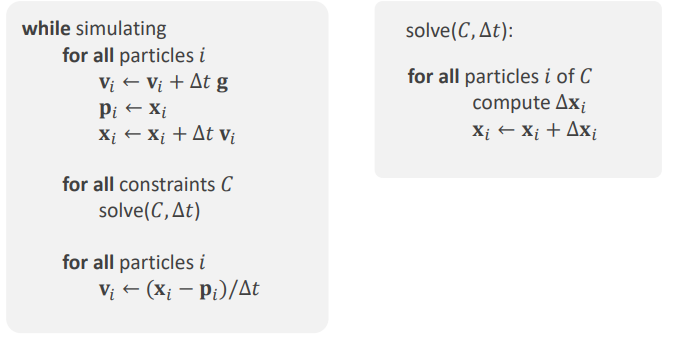## 1. 根据外力更新粒子速度位置，无需考虑粒子间关系

（如果有碰撞，也在这里处理）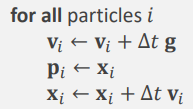## 二维情况：一个弹簧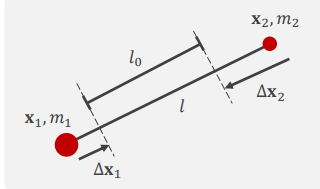C ( x 1 , x 2 ) = ∣ x 1 − x 2 ∣ − L 0 C(x1, x2) = |x1 - x2| - L0

∇ C 1 = ( x 1 − x 2 ) / ∣ x 1 − x 2 ∣ ∇ C 2 = ( x 2 − x 1 ) / ∣ x 2 − x 1 ∣ \nabla C1 = (x1 - x2) / |x1 - x2| \nabla C2 = (x2 - x1) / |x2 - x1|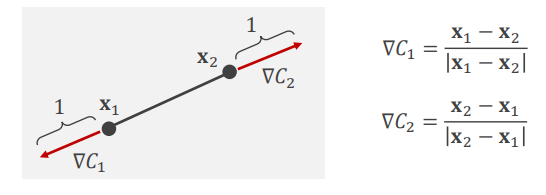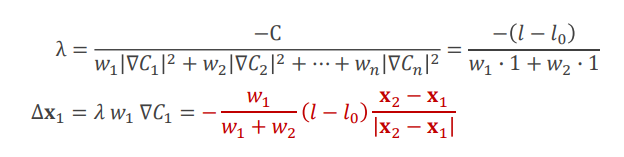## 三维情况：一个四面体

C = ( V − V 0 ) C = (V - V0)

1 6 [ ( x 2 − x 1 ) × ( x 3 − x 1 ) ] ⋅ ( x 4 − x 1 ) \frac{1}{6} [(x2 - x1)\times (x3 - x1)] \cdot (x4 - x1)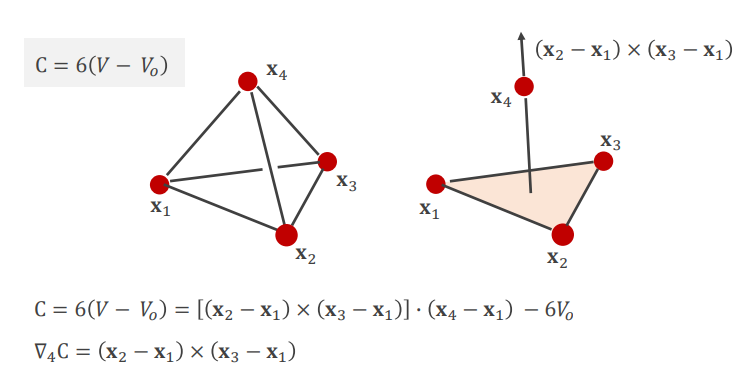g r a d C 4 = ( x 2 − x 1 ) × ( x 3 − x 1 ) gradC4 = (x2 - x1) \times (x3 - x1)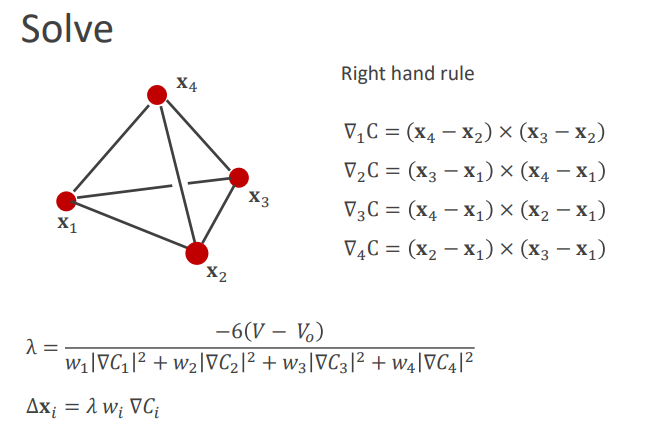# 代码

simulate函数为算法的主体，它分为三步：

1. preSolve
2. solve
3. postSolve

## preSolve

for all particles:
vel[i] += gravity * dt
prevPos[i] = pos[i]
pos[i] += vel[i] *dt
collision处理：当y<0时把pos挪到0


## solve

1. solveEdge
2. solveVolume

### solveEdge

for i in all edges:
alpha = C/dt^2
grad = pos0- pos1
s = - (L - L0)/(1/m + alpha)
pos0[i] += grad * (s/m)
pos1[i] += grad * (-s/m)


### solveVolume

for i in all tets:
alpha = C/dt^2
for j in 4:
temp0 = pos1 - pos0
temp1 = pos2 - pos0
grad[j] = (1/6) * tem0.cross(temp1)
w = sum((1/m) * ||grad[j]||^2 )
s = -(V - V0) / (w + alpha)
for j in 4:
pos[i] = grad[j] * (s/m)


## postSolve

for i in all particles:
vel[i] = (pos[i] - prevPos[i])/dt


# 代码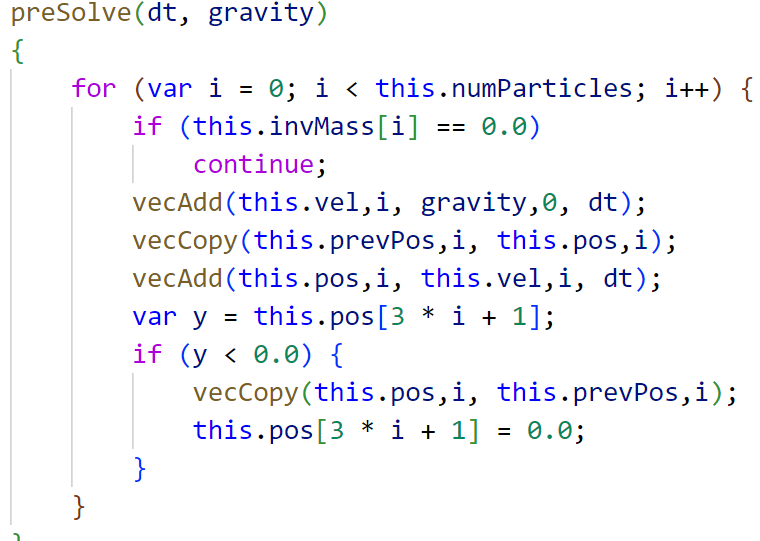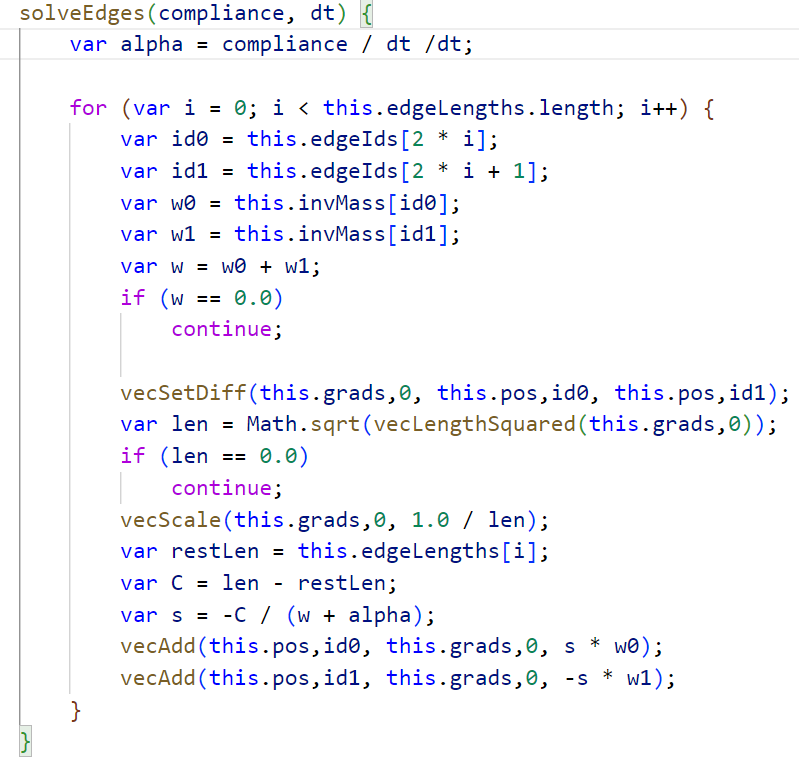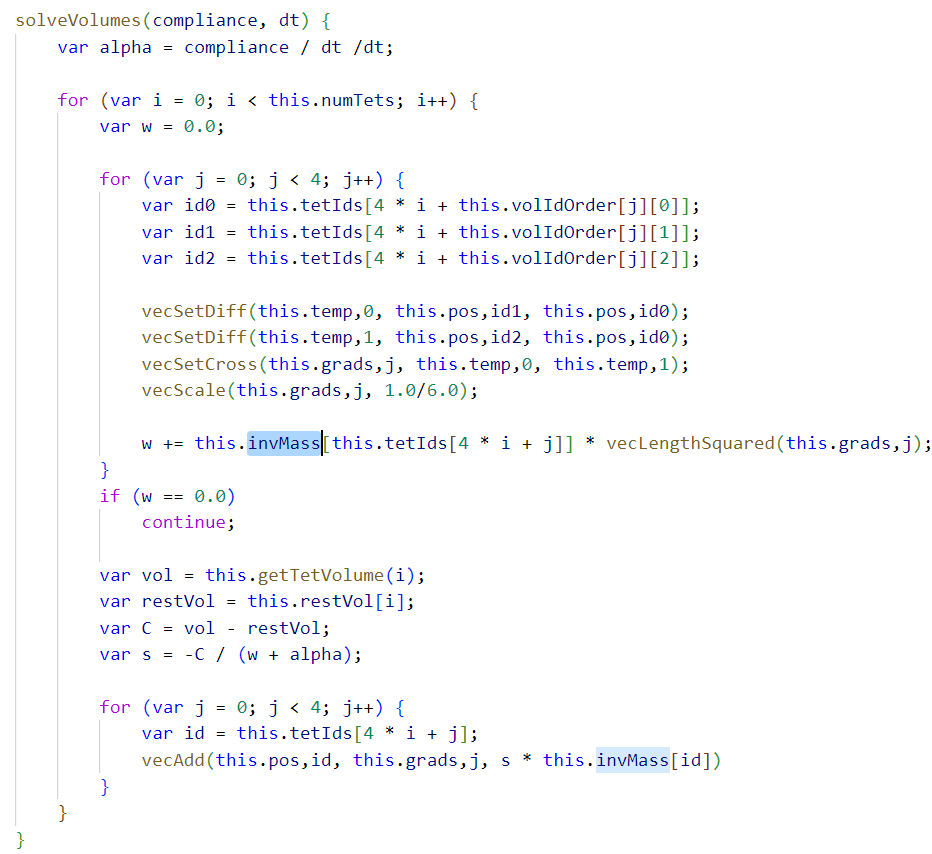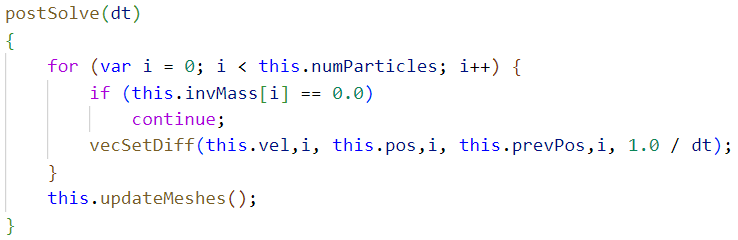08-28
07-12
07-01
11-16870
12-25909
12-22777
05-1554
04-291032
06-197万+
05-198万+
05-169万+
04-293842
06-303691

### “相关推荐”对你有帮助么？

•非常没帮助
•没帮助
•一般
•有帮助
•非常有帮助

©️2022 CSDN 皮肤主题：技术黑板 设计师：CSDN官方博客被折叠的  条评论 为什么被折叠?到【灌水乐园】发言beidou111

¥2 ¥4 ¥6 ¥10 ¥20余额支付 (余额：-- )扫码支付获取中扫码支付点击重新获取扫码支付1.余额是钱包充值的虚拟货币，按照1:1的比例进行支付金额的抵扣。
2.余额无法直接购买下载，可以购买VIP、C币套餐、付费专栏及课程。余额充值